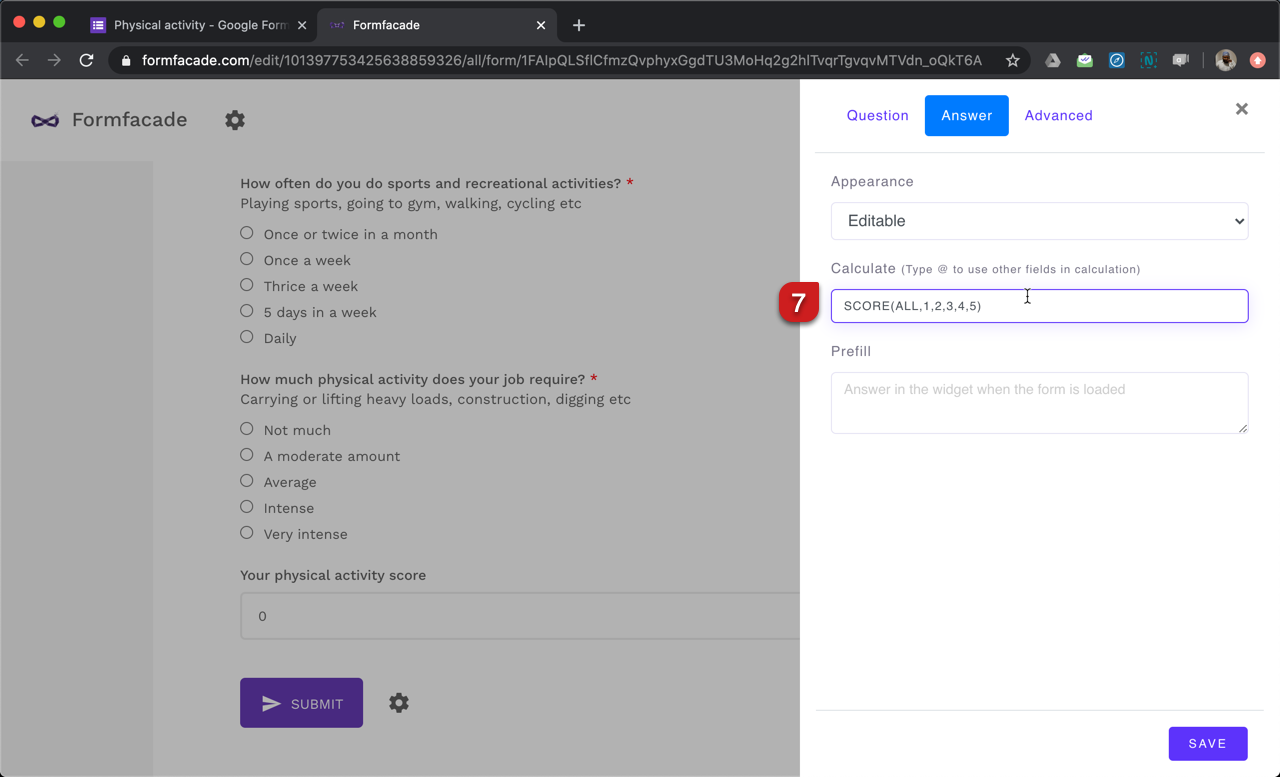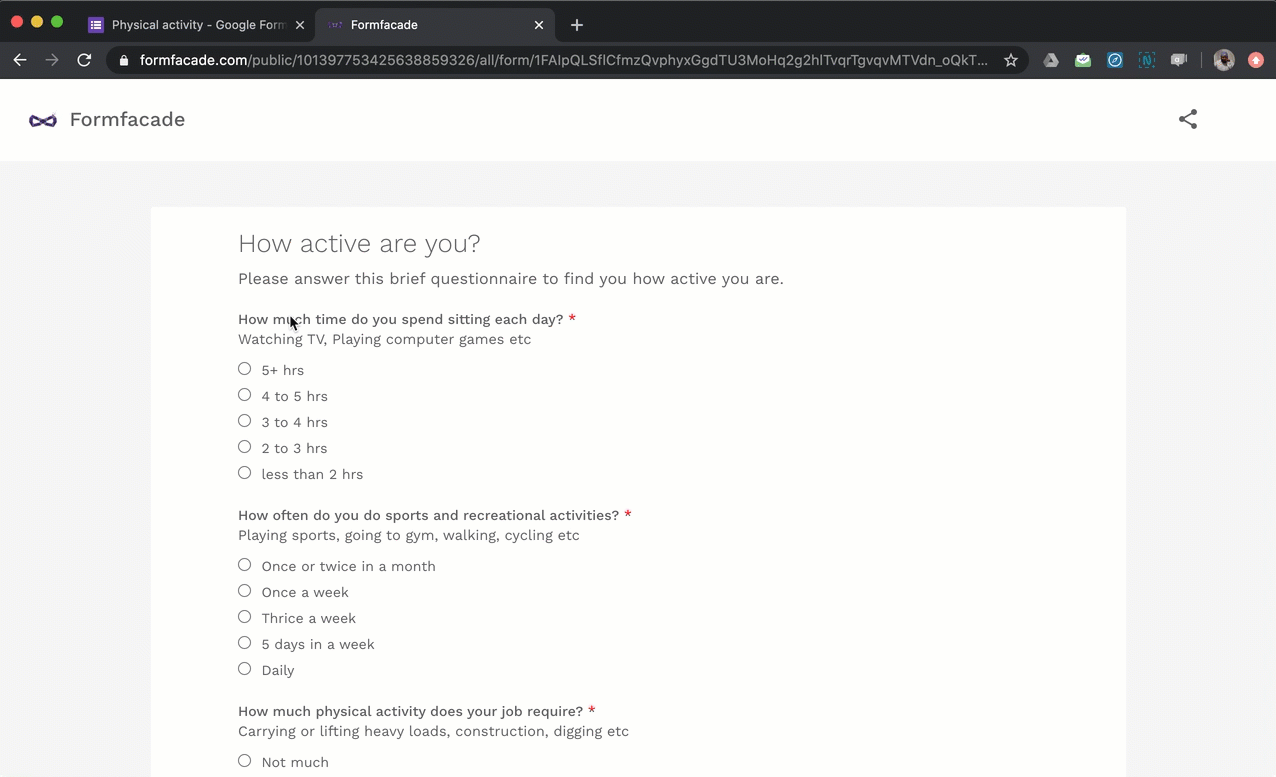### You can assign point values to questions in Google Forms by creating a quiz, but you cannot assign different points to each answer in a multiple choice question and calculate the total score. To do that, you can use the Formfacade addon for Google Forms.

Let's create a simple questionnaire with 3 multiple choice questions with five answer choices each (see below). We will calculate the total score to determine how active a person is.

How much time do you spend sitting each day?
(a) 5+ hrs
(b) 4 to 5 hrs
(c) 3 to 4 hrs
(d) 2 to 3 hrs
(e) Less than 2 hrs

How often do you do sports and recreational activities?
(a) Once or twice in a month
(b) Once a week
(c) Thrice a week
(d) 5 days in a week
(e) Daily

How much physical activity does your job require?
(a) Not much
(b) A moderate amount
(c) Average
(d) Intense
(e) Very intense

Points: We will use the same points scale for all the three multiple choice questions
Answer choice (a) - 1 point
Answer choice (b) - 2 points
Answer choice (c) - 3 points
Answer choice (d) - 4 points
Answer choice (e) - 5 points

We will calculate the total score by adding the individual points for the 3 multiple choice questions. The total score would be between 3 and 15.

#### How to assign points and calculate scores using Formfacade?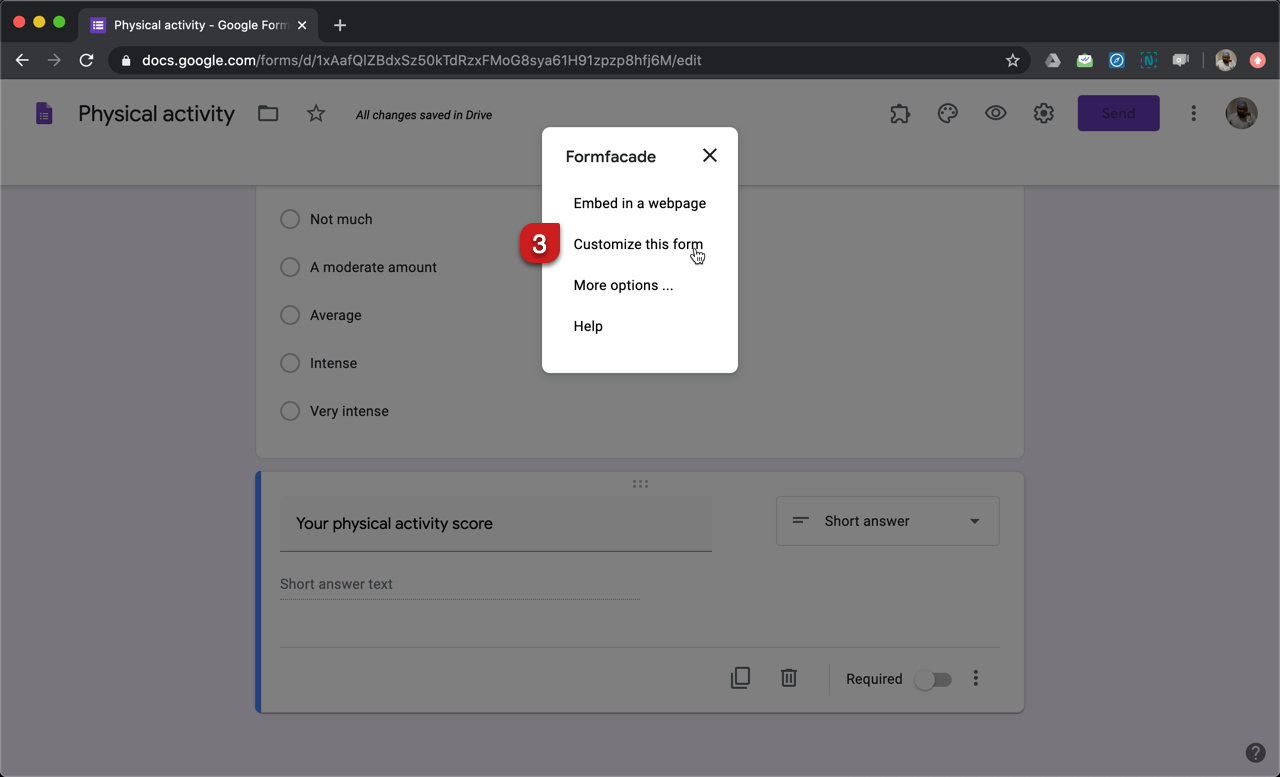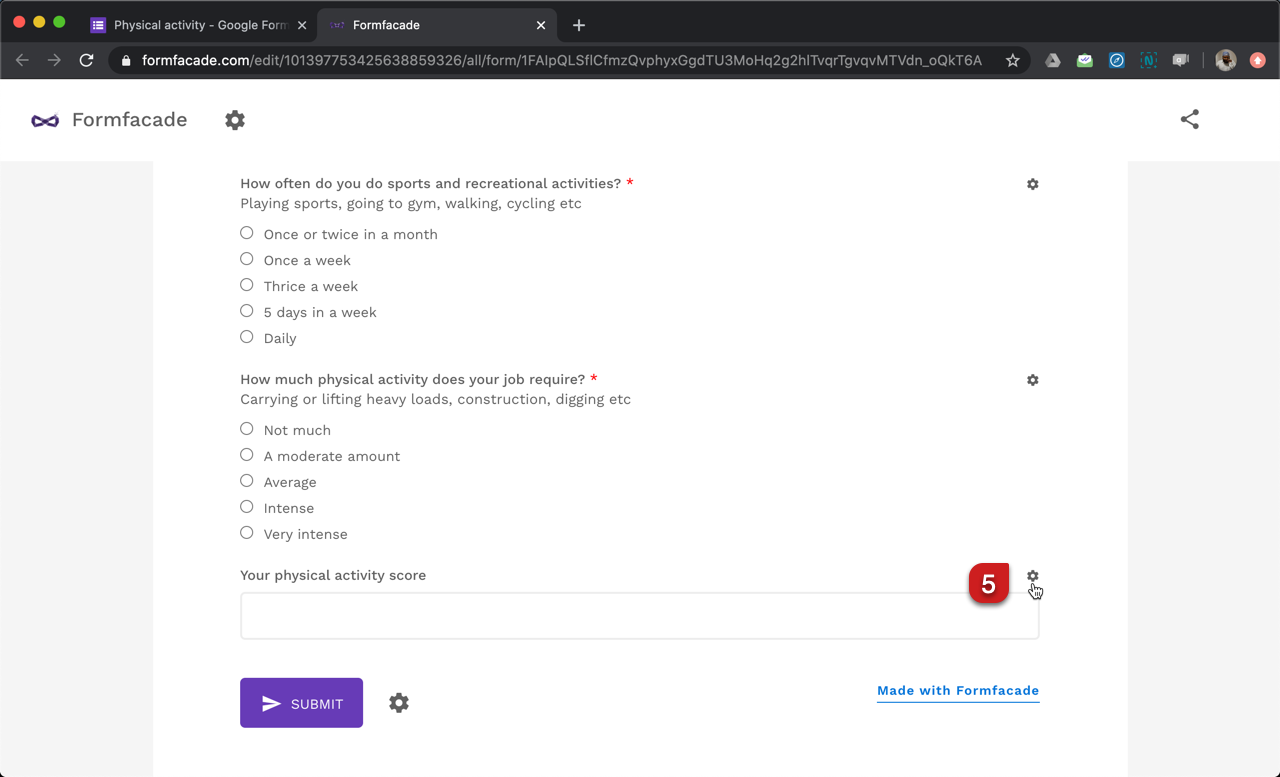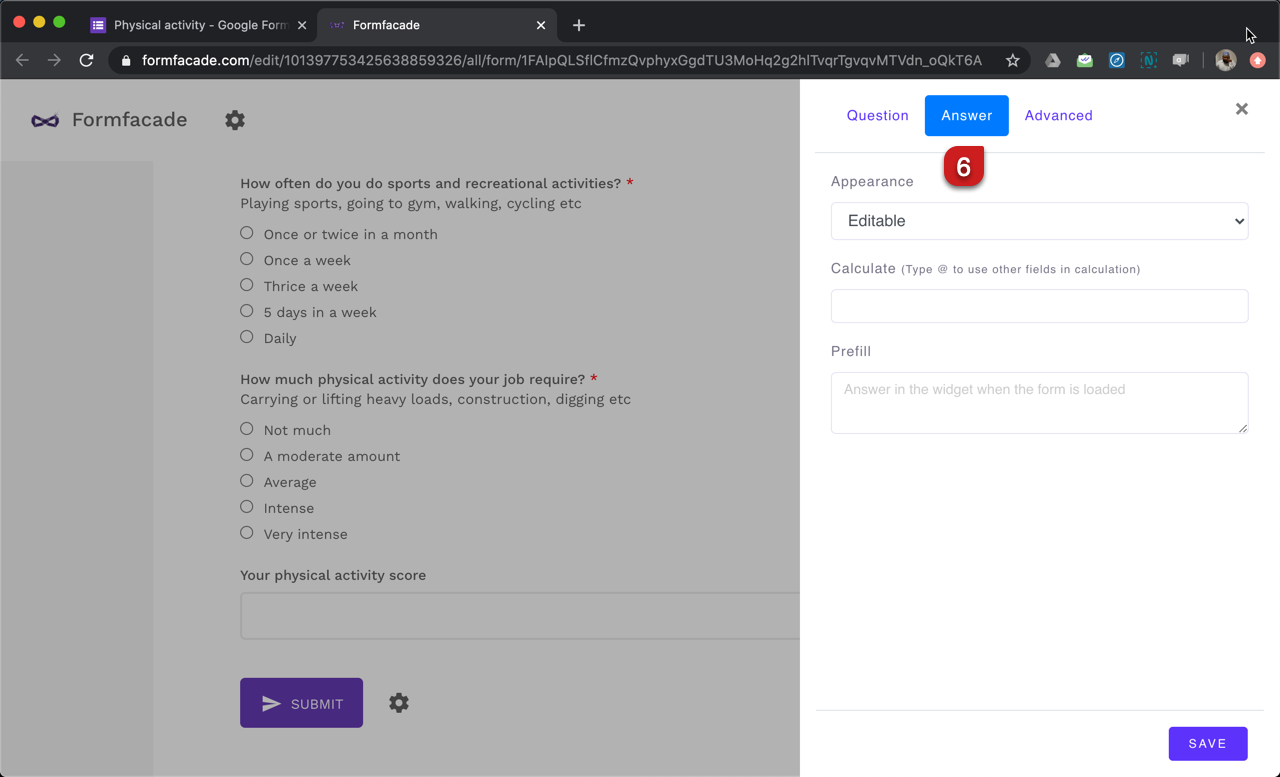#### SCORE function

SCORE() function allows you to assign scores to each answer choices in multiple choice questions, checkboxes questions and grid questions. It takes two input parameters:
1. The first parameter specifies whether the score should be calculated for all questions in the section (SECTION) or questions across all the sections (ALL) or only the questions that matches the search term in the description.
2. The second parameter is the points to be assigned for different answer choices (a), (b), (c), (d), (e) etc.

In this example, we will use SCORE(ALL,1,2,3,4,5) to calculate the physical activity score.
First parameter: ALL option to calculate the total score across the three multiple choice questions.
Second parameter: 1, 2, 3, 4, 5 to assign points for answer choices (a), (b), (c), (d), (e) respectively.